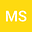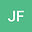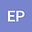General decay and blow up of solutions for a variable-exponent viscoelastic double-Kirchhoff type wave equation with nonlocal degenerate damping
•••Jorge Ferreira
Federal Fluminense University
Author ProfileErhan Pişkin
Dicle University
Author Profile## Abstract

In this paper we consider a viscoelastic double-Kirchhoff type wave equation of the form $$u_{tt}-M_{1}(\|\nabla u\|^{2})\Delta u-M_{2}(\|\nabla u\|_{p(x)})\Delta_{p(x)}u+(g\ast\Delta u)(x,t)+\sigma(\|\nabla u\|^{2})h(u_{t})=\phi(u),$$ where the functions $M_{1},M_{2}$ and $\sigma, \phi$ are real valued functions and $(g\ast\nabla u)(x,t)$ is the viscoelastic term which are introduced later. Under appropriate conditions for the data and exponents, the general decay result and blow-up of solutions are proved with positive initial energy. This study extends and improves the previous results in the literature to viscoelastic double-Kirchhoff type equation with degenerate nonlocal damping and variable-exponent nonlinearities.